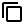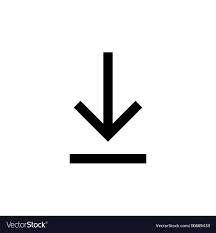# Correlation Coefficient

## Correlation Calculator - Find Correlation coefficient between 2 variables using Pearson correlation formula (R). Generate Scatter Plot using the given set of data

HomeMath

Correlation Calculator

Find Correlation Coefficient between the 2 variables. Enter your data as comma separated values or with spaces. You can also import csv or any data file with delimiters## Correlation Statistics## XY Scatter Plot

### Data shows Strong Positive Correlation## Frequently Asked Questions on Correlation Coefficient Calculator